# VFX Graph and Vortices

Hello everyone, my name is Grigory Dyadichenko and I love VFX. In this article, I would like to talk more about VFX Graph, about its functions and how you can make simple vortices there (in various ways). If you are interested in the topic of Unity and VFX, welcome to cat.

I must say right away that this article is more about working with VFX Graph than about creating a vortex itself. From two points of view. If you need to make a beautiful tornado, there are better and better ways. As an example one way is described here and it will often be more optimal, simpler and more effective. On the other hand, I will not discuss “vortex simulation”, the Navier-Stokes equations, or the Kelvin circulation theorem. We will analyze how to make an effect similar to a whirlwind, what tools are available for this in VFX Graph, etc. This kind of work with particles can rather complement the effect from the video above, or make an impact on the environment like tornadoes through particles. Well, for starters, let’s show what happened.

## Count preparation

Unlike Shuriken in VFX Graph, shape emitters do not equalize speed with that shape. If we want to make a la tornado, the first thing we need to do is an emitter in the form of a cone. There is one in VFX Graph.

Also, replacing the standard particles with softer ones and increasing the number of particles, you get something like this effect. For convenience, we also need to control the angle of our vortex and the height. You can of course do this directly through the radii and parameters of the cone, but the angle is a more understandable metric.

The cone is essentially a “twisted” triangle. If we disassemble the triangle, then in our case it consists of Top Raduis and Height. At the same time, it is known from mathematics that the tangent of an angle is equal to the ratio of the opposite side to the adjacent side in a right triangle. Since we need to set the height and angle, it remains to find the Top Radius. You can see the calculation in the picture below.

That is, the radius is equal to the tangent of the angle at the base, multiplied by the height. Let’s bring this all to the VFX Graph.

Since it is more customary to specify angles in degrees, not in radians, but in tangent, the angle is in radians, we first convert degrees to radians using the formula: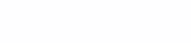But since it is more convenient for me to use the general angle from the base of the cone, we divide it by 2 or divide it by 360 instead of 180. Everything is placed in the graph in external parameters for ease of control, now we can adjust our cone by angle and height.

Also in the VFX Graph we can control particle spawning. In general, in order for the density of vortex particles not to change (up to a certain limit) when changing the height and angle, we need to change the spawn rate depending on the parameters. Since I want the particles to be on the lateral surface of the cone, it is logical to use the area of ​​the lateral surface of the cone as a parameter. The formula for the side surface of a cone is: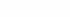so now we need to find l. There are several ways to do this, as an example of the Pythagorean theorem, but we will use a simpler one, since we have an angle, and even in radians. As is known from the same trigonometry: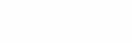in our terms. Therefore, the desired: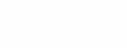We write this as a graph with the formula for the lateral surface of the cone:

So, with the spawn, we sort of figured it out. Yes, it’s worth saying that the math above is fine to get a uniform distribution of particles on the surface of a cone. For the distribution of particles in a volume, a volume formula is needed. The fact that particles spawn on the surface is set in the settings Set Position (Shape: Arc Cone). Both the additional constant in the graph and the Rate parameter are just for convenience.

## Path 1. Tangent to the circle

It’s time to start dealing with speeds. The first idea that came to my mind was to get the desired effect. Tangent to the circumference of a cone. When viewed from above, the cone is just a series of nested circles. We can always find out the position of a particle in the VFX Graph with Get Attribute: position, and then it’s all a matter of technique. Since we have a local coordinate system, the tangent to the circle we need is the normal to the radius. Normal in our case is: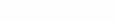Since we are in the XZ plane. But in the local coordinate system, we will also take as a simplification that all particles rotate around the (0, 0) axis. So dx = x – 0 = x, where x is the position of a particular particle. That is: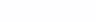What in the graph will turn into

and looks something like this:

It looks good in general, but this method has one problem. If we try to add the speed of rotation of the particles, then the particles will start entering new orbits purely because of the principle of the solution. For example, particle rotation speed 5:

If that speed is done simply by multiplying the speed vector by a constant.

## Path 2. Particle rotation

Well, that didn’t work out. Let’s try something else. Here I will probably immediately show the graph in its entirety, and then I will explain what it does and why:

This whirlwind looks like this:

The idea is that we are now working not in speed, but in position. We have a central axis and around it in the XZ plane we rotate the particles. Therefore, it is important that the position is now set, and not the speed. The speed depends on the radius of the circle so that the particles move faster if they are in a larger orbit + added a little randomness for a more “natural” effect. The upper part with the emission of particles has not changed in any way, in fact, we have changed only the principle of movement. Visually, in general, it’s not bad and you can change the speed, while not a single particle will leave the orbit. But then I decided to add another “orbit tilt” function and the graph became like this: